Next: Long Time Observables Up: Methods of quality assessment Previous: Model systems and test

## Short Time Observables

To measure the numerical accuracy of the force approximation we follow the suggestion in ref. . We consider the root mean square (rms) error of forces,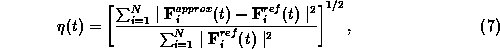where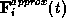and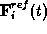denote the approximated and the exact Coulomb force, respectively, acting on atom i at time t, and where the forces are calculated using the trajectory of the reference simulation. As an accuracy measure we take the meanof the rms errorfor the simulated trajectory. The rms error of FAMUSAMMwill fluctuate in the course of a simulation, because, as is apparent from Figure 4, the accuracy of the force approximation should vary from step to step. The size of these fluctuations is measured by the standard deviation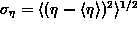.

Taking the point of view of numerical mathematics one might expect thatand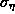represent measures for the algorithmic noise associated with a given approximation method. However, as discussed in refs.  and  there are physical considerations contradicting that expectation. For instance, the DC-1d extrapolation scheme has been designed to guarantee optimal energy conservation and other useful properties. Correspondingly, that method has been demonstrated to entail smaller algorithmic noise in the framework of pure force extrapolation than the linear extrapolation scheme, although it exhibits larger values ofandthan the latter [16, 20]. It remains to be checked whether this is also the case in our framework of local Taylor expansion extrapolations. Therefore we also have to consider the size of uncontrolled algorithmic noise. In the microcanonical simulations at hand the estimation of that size is trivial. As algorithmic noise is the sole cause for energy transfer into such systems, one simply has to monitor the drift of the total energy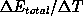.

Helmut Grubmueller
Wed Apr 30 15:40:09 MET DST 1997